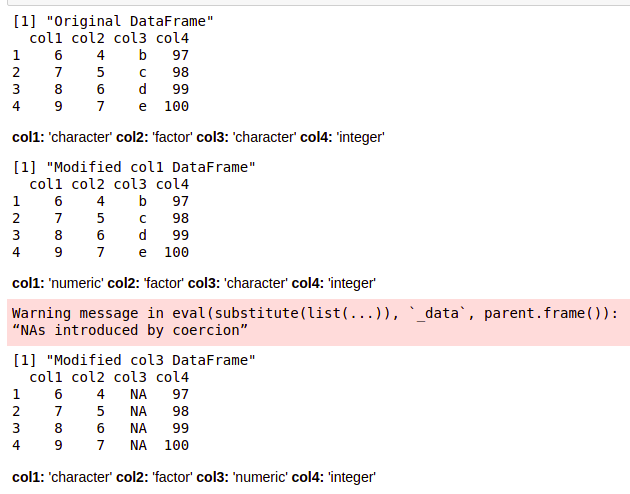Open In App

# How to convert DataFrame column from Character to Numeric in R ?

In this article, we will discuss how to convert DataFrame column from Character to Numeric in R Programming Language.

All dataframe column is associated with a class which is an indicator of the data type to which the elements of that column belong to. Therefore, in order to simulate the data type conversion, the data elements have to be converted to the desired data type in this case, that is all the elements of that column should be eligible to become numerical values.

Note: sapply() method can be used to retrieve the data type of the column variables in the form of a vector.

Method 1 : Using transform() method

The character type columns, be single characters or strings can be converted into numeric values only if these conversions are possible. Otherwise, the data is lost and coerced into missing or NA values by the compiler upon execution.

This approach depicts the data loss due to the insertion of missing or NA values in place of characters. These NA values are introduced since interconversion is not directly possible.

## R

 `# declare a dataframe``# different data type have been``# indicated for different cols``data_frame <- ``data.frame``(``               ``col1 = ``as.character``(6 : 9),``               ``col2 = ``factor``(4 : 7),``               ``col3 = ``letters``[2 : 5],``               ``col4 = 97 : 100, stringsAsFactors = ``FALSE``)` `print``(``"Original DataFrame"``)``print ``(data_frame)` `# indicating the data type of``# each variable``sapply``(data_frame, class)` `# converting character type``# column to numeric``data_frame_col1 <- ``transform``(data_frame,``                             ``col1 = ``as.numeric``(col1))``print``(``"Modified col1 DataFrame"``)``print ``(data_frame_col1)` `# indicating the data type of``# each variable``sapply``(data_frame_col1, class)` `# converting character type column``# to numeric``data_frame_col3 <- ``transform``(data_frame,``                             ``col3 = ``as.numeric``(col3))``print``(``"Modified col3 DataFrame"``)``print ``(data_frame_col3)` `# indicating the data type of each``# variable``sapply``(data_frame_col3, class)`

Output:Explanation: Using the sapply() method, the class of the col3 of the dataframe is a character, that is it consists of single-byte character values, but on the application of transform() method, these character values are converted to missing or NA values, because the character is not directly convertible to numeric data. So, this leads to data loss.

The conversion can be made by not using stringAsFactors=FALSE and then first implicitly converting the character to factor using as.factor() and then to numeric data type using as.numeric(). The information about the actual strings is completely lost even in this case. However, the data becomes ambiguous and may lead to actual data loss. The data is simply assigned numeric values based on the lexicographic sorting result of the column values.

## R

 `# declare a dataframe``# different data type have been``# indicated for different cols``data_frame <- ``data.frame``(``               ``col1 = ``as.character``(6 : 9),``               ``col2 = ``factor``(4 : 7),``               ``col3 = ``c``(``"Geeks"``, ``"For"``, ``"Geeks"``, ``"Gooks"``),``               ``col4 = 97 : 100)``print``(``"Original DataFrame"``)``print ``(data_frame)` `# indicating the data type of``# each variable``sapply``(data_frame, class)` `# converting character type column``# to numeric``data_frame_col3 <- ``transform``(data_frame,``                             ``col3 = ``as.numeric``(``as.factor``(col3)))``print``(``"Modified col3 DataFrame"``)``print ``(data_frame_col3)` `# indicating the data type of each``# variable``sapply``(data_frame_col3, class)`

Output:

``` "Original DataFrame"
col1 col2  col3 col4
1    6    4 Geeks   97
2    7    5   For   98
3    8    6 Geeks   99
4    9    7 Gooks  100
col1      col2      col3      col4
"factor"  "factor"  "factor" "integer"
 "Modified col3 DataFrame"
col1 col2 col3 col4
1    6    4    2   97
2    7    5    1   98
3    8    6    2   99
4    9    7    3  100
col1      col2      col3      col4
"factor"  "factor" "numeric" "integer"```

Explanation: The first and third-string in col3 are the same therefore, assigned the same numeric value. And in total, the values are sorted in ascending order and then assigned corresponding integer values. “For” is the smallest string appearing in lexicographic order, therefore, assigned numeric value of 1, then “Geeks”, both instances of which are mapped to 2 and “Gooks” is assigned a numeric value of 3. Thus, the col3 type changes to numeric.

Method 2 : Using apply() method

The apply() method in R allows the application of a function over multiple columns together. The function may be user-defined or inbuilt, depending upon user’s need.

Syntax: apply ( df , axis , FUN)

Arguments :

• df – The dataframe to apply the function on
• axis – The axis to apply the function upon
• FUN- User-defined method to apply

Example:

## R

 `# declare a dataframe``# different data type have been``# indicated for different cols``data_frame <- ``data.frame``(``               ``col1 = ``as.character``(6:9),``               ``col2 = ``as.character``(4:7),``               ``col3 = ``c``(``"Geeks"``,``"For"``,``"Geeks"``,``"Gooks"``),``               ``col4 = ``letters``[1:4])` `print``(``"Original DataFrame"``)``print ``(data_frame)` `# indicating the data type of each``# variable``sapply``(data_frame, class)` `# defining the vector of columns to``# convert to numeric``vec <- ``c``(1,2)` `# apply the conversion on columns``data_frame[ , vec] <- ``apply``(data_frame[ , vec,drop=F], 2,          ``                    ``function``(x) ``as.numeric``(``as.character``(x)))``print``(``"Modified DataFrame"``)``print ``(data_frame)` `# indicating the data type of each variable``sapply``(data_frame, class)`

Output:

``` "Original DataFrame"
col1 col2  col3 col4
1    6    4 Geeks    a
2    7    5   For    b
3    8    6 Geeks    c
4    9    7 Gooks    d
col1     col2     col3     col4
"factor" "factor" "factor" "factor"
 "Modified DataFrame"
col1 col2  col3 col4
1    6    4 Geeks    a
2    7    5   For    b
3    8    6 Geeks    c
4    9    7 Gooks    d
col1      col2      col3      col4
"numeric" "numeric"  "factor"  "factor" ```

Explanation: The col1 and col2 types are converted to numeric. However, this method is applicable to pure numeric data converted to character. It throws an error “NAs introduced by coercion” upon execution for col3 and col4.